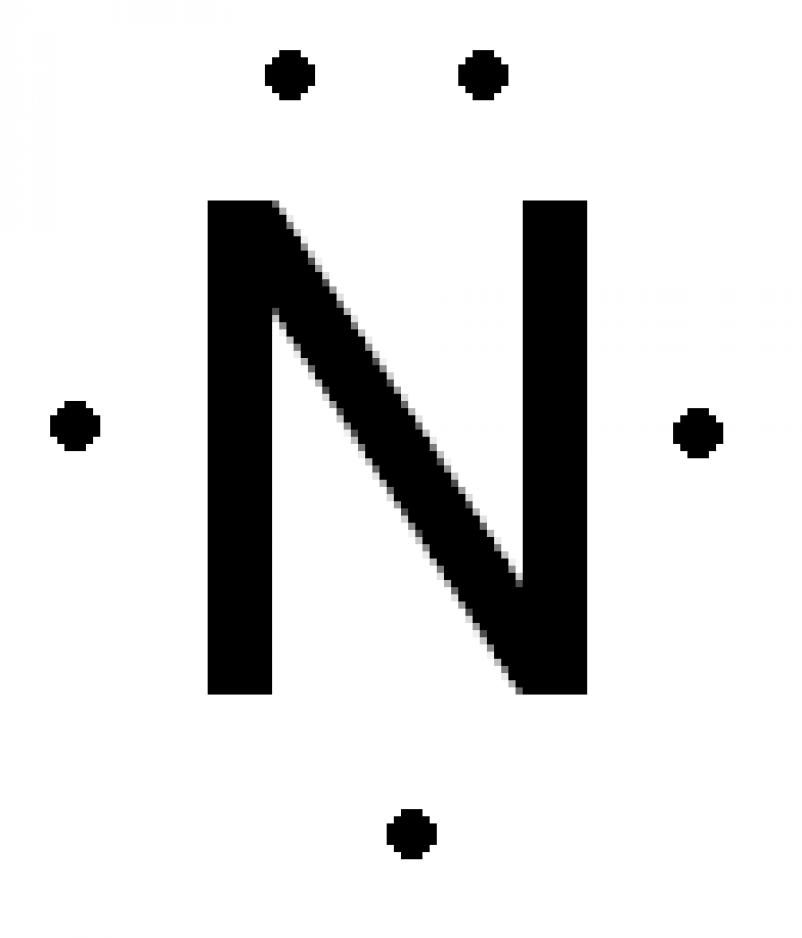# Is this molecule polar?

### Disclaimer

It is bad practice to draw out your molecules in 2 dimensions because that is not how they are. I chose to do this in 2D because it is easier to explain.

Note that the bond angles are wrong too: the angle in CH4 is 109.5°, not 90° as I said and the bond angle in NH4 is 107°. BHCl2 does have a bond angle of 120° and is 2D, however.

## Challenge

Given the formula of a simple molecule as input, output a truthy value if the molecule is polar (asymmetrical) or a falsey value if the molecule is non-polar (symmetrical).

## Determining Polarity

To determine whether a molecule is polar or not, we will have to look at its shape. For the purposes of this challenge, we will assume that molecules are 2D.

To demonstrate how to determine the polarity of a molecule, let us use three examples: methane (CH4), ammonia (NH3) and BHCl2.

### CH4

Firstly, lets take the centre molecule, carbon. Carbon is in group four of the periodic table so it has four outer shell electrons, as shown below:Now, we add the hydrogen atoms (each hydrogen atom supplies its own electron to make a pair):Since there are no lone pairs of electrons, we can draw it with 90° between each bond since all bonds repel equally:We can now see that methane is symmetrical so it is non-polar.

### NH3

Like above, we take the centre molecule, nitrogen and draw out its outer shell electrons:Adding the hydrogen atoms, we notice that there is a lone pair of electrons:Since the lone pair is closer to the atom, it repels more than a bonding pair, so the angle between the bonds is smaller:Evidently, NH3 is not symmetrical, so it is polar.

### BHCl2

Taking boron as the centre molecule, we draw the three outer electrons:We then add the hydrogen and the chlorines:There are no lone pairs, so the bonds repel equally, forming an angle of 120°:Although, the shape is symmetrical, the hydrogen atom makes the molecule asymmetrical, so BHCl2 is polar.

## Rules

Built-ins which access molecule information are disallowed. You may access periodic table data using built-ins or without adding the file to your byte count. This data may be stored however you wish.

Input will only ever be a valid simple molecule: a single central atom (p-block element) which comes first in the formula and up to eight outer atoms (hydrogen, fluorine, chlorine, bromine, iodine, astatine) which only use single bonds.

You will never get a polyatomic ion or a molecule with co-ordinate (dative covalent) bonds as input. For example, with NH4, there would be a single unpaired electron, so the only for NH4 to form a stable molecule would be to lose the extra electron and become an ion (a polyatomic ion, since there is more than one atom in the ion).

If there are multiple lone pairs, they have to be spaced as far as possible from each other. For example, two lone pairs will have to be opposite from each other.

If there are non-polar and polar isomers of a molecule (e.g. SiF2Cl2) you should assume the molecule is non-polar.

## Examples

Input -> Output
OH2 -> Falsey
CCl4 -> Falsey
OF4 -> Truthy
CH4 -> Falsey
NH3 -> Truthy
BHCl2 -> Truthy


## Winning

Shortest code in bytes wins.

• So, have I got the right idea if I say: we must return truthy if any of the counts of "outer" atoms does not divide the total number of outer atoms and otherwise return truthy iff the number of electrons for the "central" atom minus the total number of "outer" atoms divided by two is odd? – Jonathan Allan May 20 '17 at 11:55
• @Jonathan Yes, I think that's an easy way to work it out – Beta Decay May 20 '17 at 11:58
• actually I don't think "if any of the counts of "outer" atoms does not divide the total number of outer atoms" is right, for example OH2Cl4 (or Cl4H2O as it would normally be called) has 6 outer atoms, but the count of Cl, 4, does not divide 6. – Jonathan Allan May 20 '17 at 12:37
• The way you went from a hi-res diagram for Carbon, a lower-res diagram for Nitrogen and finally hand-written diagrams made me think about this Smurf joke :-p (In French, sorry, but you get the idea.) – Arnauld May 20 '17 at 12:43
• can periodic table data (not counted as part of score) be given as a dictionary, string, or array? accessing a file can take a lot of bytes in some languages. – Level River St May 21 '17 at 1:34

# Python 3, 296 bytes

import P,re,itertools
r="[A-Z][a-z]?"
f=re.findall(r,re.sub("(%s)(\d)"%r,lambda g:g.group(1)*int(g.group(2)),input()))
print(all(any(any(q[:len(q)//i]*i==q for i in range(2,len(q)))for q in[j[i:]+j[:i]for i in range(len(j))])<1for j in itertools.permutations(f+['e']*-(len(f)-P.a[f.pop(0)]>>1))))


Try it online!

-29 bytes thanks to ovs

Prints False or True.

This requires a dict of all p-block element symbols to the number of valence electrons they have. H is not necessary since it will only be one of the surrounding atoms. The dict is stored in P.py as a variable named a (it's written in the input for TIO and then placed into a Python file by the header)

• 303 bytes – ovs Oct 25 '17 at 14:27
• 296 bytes – ovs Oct 25 '17 at 14:42